Courses

# CAT Previous Year Questions: Number Series

## 20 Questions MCQ Test Logical Reasoning (LR) and Data Interpretation (DI) | CAT Previous Year Questions: Number Series

Description
This mock test of CAT Previous Year Questions: Number Series for Banking Exams helps you for every Banking Exams entrance exam. This contains 20 Multiple Choice Questions for Banking Exams CAT Previous Year Questions: Number Series (mcq) to study with solutions a complete question bank. The solved questions answers in this CAT Previous Year Questions: Number Series quiz give you a good mix of easy questions and tough questions. Banking Exams students definitely take this CAT Previous Year Questions: Number Series exercise for a better result in the exam. You can find other CAT Previous Year Questions: Number Series extra questions, long questions & short questions for Banking Exams on EduRev as well by searching above.
QUESTION: 1

### Wrong Number Series: 59, 41, 68, 33, 77, 23

Solution: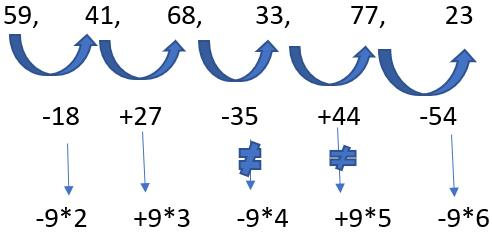Therefore, wrong no. is 33

QUESTION: 2

### 9, 5, 6, 10.5, 23, 90, 183,

Solution:

The solution is:
(9 + 1) * 0.5 = 5
(5 + 1) * 1 = 6
(6 + 1) * 1.5 = 10.5
(10.5 + 1) * 2 = 23
(23 + 1) * 2.5 = 60 ≠ 90
(60 + 1) * 3 = 183

Therefore, wrong no. is 90.

QUESTION: 3

### 318, 168, 78, 38, 18, 8, 3

Solution:

The solution is:
(3 + 1) * 2 = 8
(8 + 1) * 2 = 18
(18 + 1) * 2 = 38
(38 + 1) * 2 = 78
(78 + 1) * 2 = 158 ≠ 168
(158 + 1) * 2 = 318

Therefore, wrong no. is 168

QUESTION: 4

3, 8, 18, 38, 80, 158, 318

Solution:

The solution is:
3 * 2 + 2 = 8
8 * 2 + 2 = 18
18 * 2 + 2 = 38
38 * 2 + 2 = 78 ≠  80
78 * 2 + 2 = 158
158 * 2 + 2 = 318
Therefore, wrong no. is 80

QUESTION: 5

120, 143, 168, 195, 225, ?

Solution:

(11)*11 – 1 = 120
11*13 = 143
13*13-1 = 168
13*15 = 195
15*15-1=225
15*17=255
Answer is 255 so, none of these.

QUESTION: 6

32, 39, 65, 128, 253, 467

Solution: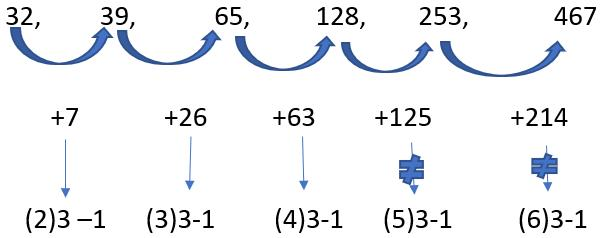Therefore, wrong no. is 253

QUESTION: 7

33, 39, 56, 85, 127, 185, 254

Solution: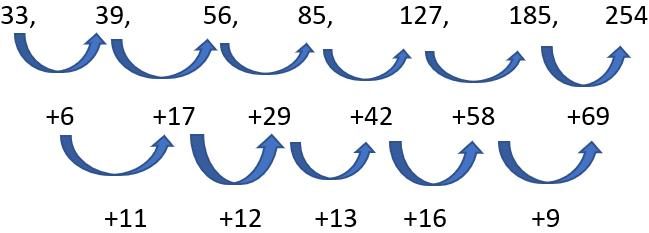Therefore, it must be 42+14 = 56   i.e. 127+56 = 183
56+ 15 = 71  i.e. 183+ 71 = 254
Hence, wrong no. is 185

QUESTION: 8

7, 9, 21, 67, 275, 1371, 8233

Solution:

The solution is :
7 * 1 + 2 = 9
9 * 2 + 3 = 21
21 * 3 + 4 = 67
67 * 4 + 5 = 273  ≠    275
273 * 5 + 6 = 1371
1371 * 6 + 7 = 8233
Therefore, wrong no. is 275

QUESTION: 9

3, 6, 15, 45, 157, 630, 2835

Solution:

The solution is :
3 * 2 = 6
6 * 2.5 = 15
15 * 3 = 45
45 * 3.5 = 157.5   ≠      157
157.5 * 4 = 630
630 * 4.5 = 2835
Therefore, wrong no. is 157

QUESTION: 10

5, 23, 43, 66, 94, 131

Solution: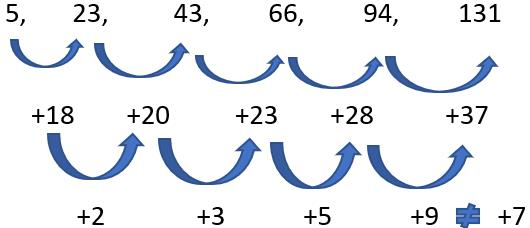Because, difference of the difference is prime numbers in increasing order . therefore, wrong no. is 131

QUESTION: 11

4, 2, 6, 0, 8, -4, 10

Solution: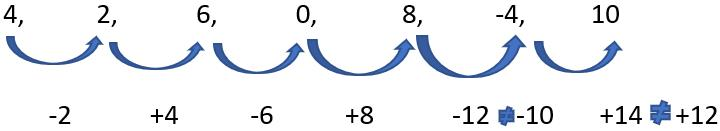Therefore, wrong no. is -4

QUESTION: 12

2478, 819, 257, 84, 24, 5

Solution:

2478/3 – 7 = 819
819/3 – 6  = 267  ≠    257
267/3 – 5 = 84
84/3 – 4 = 24
24/3 – 3 = 5
Therefore, the wrong no. is 257

QUESTION: 13

3, 11, 34, 69, 131, 223

Solution:

The solution is :
(1)3 + 2 = 3
(2)3 + 3 = 11
(3)3 + 4 = 31  ≠   34
(4)3 + 5 = 69
(5)3 + 6 = 131
Therefore, the wrong no. is 34

QUESTION: 14

9, 5, 9,  25, 95, 414

Solution:

The solution is :
9 * .5 + .5 = 5
5 * 1.5 + 1.5 = 9
9 * 2.5 + 2.5 = 25
25 * 3.5 + 3.5 = 91  ≠ 95
91 * 4.5 + 4.5 = 414
Therefore wrong no. is 95

QUESTION: 15

8, 18, 64, 272, 1395, 8424, 59045

Solution: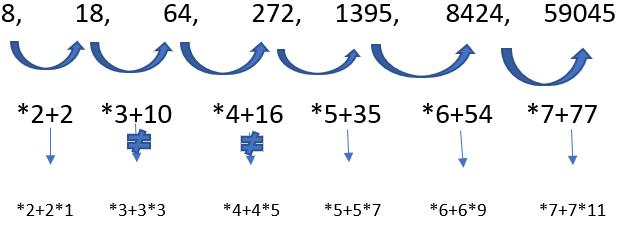Therefore, wrong no. is 64

QUESTION: 16

47, 44, 45, 46, 33, 57, 3, 88

Solution: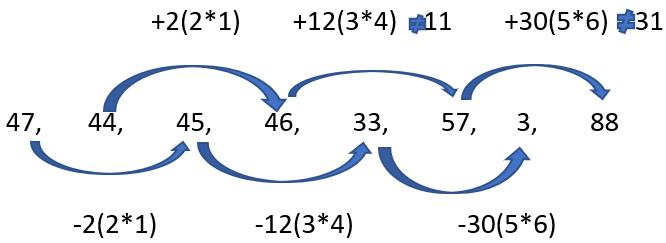Therefore, wrong no. is 57

QUESTION: 17

1, 8, 66, 460, 2758, 13785, 55146

Solution:

The solution is :
1 * 9 – 1 = 8
8 * 8 + 2 = 66
66 * 7 - 3 =459 ≠   460
459 * 6 + 4 = 2758
2758 * 5 – 5 = 13785
13785 * 4 + 6 = 55146
Therefore, wrong no. is 460

QUESTION: 18

3, 7.5, 15,  37.5, 75, 167.5, 375

Solution:

The solution is :
3 * 2.5 = 7.5
15 * 2.5 = 37.5
75 * 2.5 = 187.5   ≠  167.5
7.5 * 2 = 15
37.5 * 2 = 75
187.5 * 2 = 375
Therefore, wrong no. is 167.5

QUESTION: 19

4, 12, 42, 196, 1005, 6066, 42511

Solution:

The solution is:
4 * 2 + (2)2 = 12
12 * 3 + (3)2 = 45   ≠  42
45 * 4 + (4)2 = 196
196 * 5 + (5)2 = 1005
1005 * 6 + (6)2 = 6066
6066 * 7 +(7)2 = 2511
Therefore, wrong no. is 42

QUESTION: 20

9, 7, 15, 44, 175, 874

Solution:

The solution is :
9 * 1 – 1 = 8  ≠ 7
8 * 2 – 1 = 15
15 * 3 – 1 = 44
44 * 4 – 1 = 175
175 * 5 – 1 = 874
Therefore, wrong no. is 7